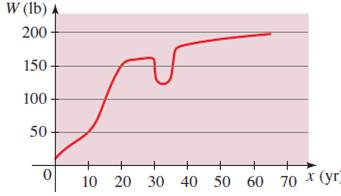# the intervals on which function is increasing or decreasing### Precalculus: Mathematics for Calcu...

6th Edition
Stewart + 5 others
Publisher: Cengage Learning
ISBN: 9780840068071### Precalculus: Mathematics for Calcu...

6th Edition
Stewart + 5 others
Publisher: Cengage Learning
ISBN: 9780840068071

#### Solutions

Chapter 2.3, Problem 45E

a.

To determine

## the intervals on which function is increasing or decreasing

Expert Solution

The intervals is increasing on (030)&(3570) or decreasing on (3035)

### Explanation of Solution

Given information : The provided graph shown belowThe graph shows that at point (030)&(3570) the graph is increasing or at point (3035) the graph is decreasing

b.

To determine

### What happen when the person was at 30 years old

Expert Solution

In the age of 30 years old the weight decreased very fast

### Explanation of Solution

Given information : The provided graph shown belowAt the age of 30 years old the person be in health problem due to this the weight decreased very fast

### Have a homework question?

Subscribe to bartleby learn! Ask subject matter experts 30 homework questions each month. Plus, you’ll have access to millions of step-by-step textbook answers!